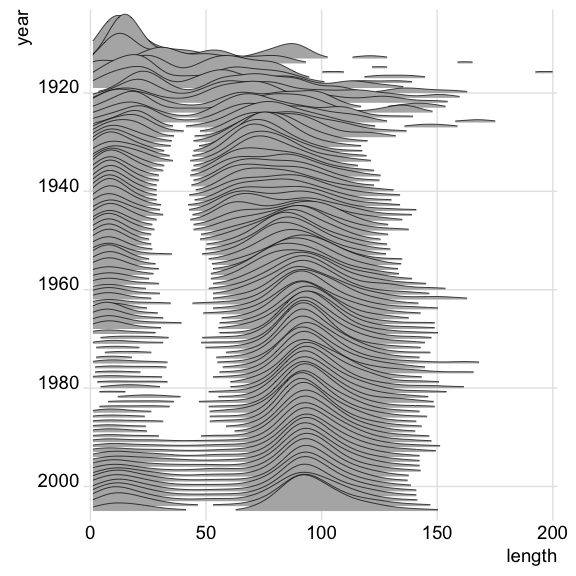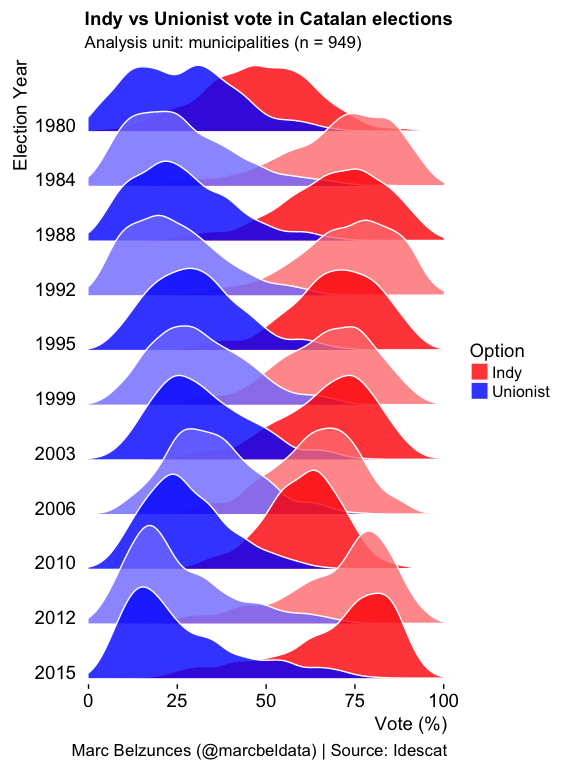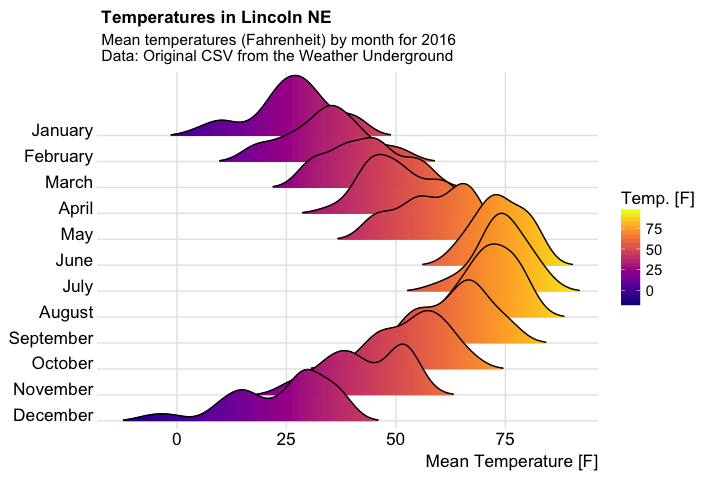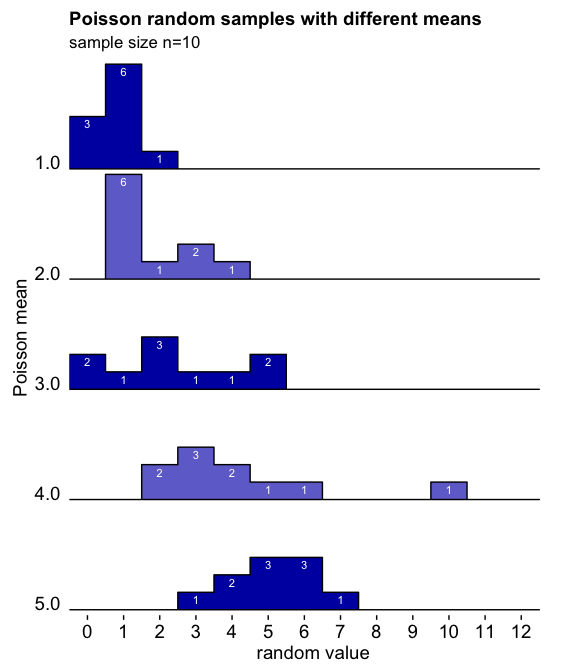# Gallery of ggridges examples

## Evolution of movie lengths over time

Data from the IMDB, as provided in the ggplot2movies package.

library(ggplot2movies)
ggplot(movies[movies$year>1912,], aes(x = length, y = year, group = year)) + geom_density_ridges(scale = 10, size = 0.25, rel_min_height = 0.03) + theme_ridges() + scale_x_continuous(limits=c(1, 200), expand = c(0.01, 0)) + scale_y_reverse(breaks=c(2000, 1980, 1960, 1940, 1920, 1900), expand = c(0.01, 0))## Results from Catalan regional elections, 1980-2015 Modified after a figure originally created by Marc Belzunces. library(tidyverse) library(forcats) Catalan_elections %>% mutate(YearFct = fct_rev(as.factor(Year))) %>% ggplot(aes(y = YearFct)) + geom_density_ridges(aes(x = Percent, fill = paste(YearFct, Option)), alpha = .8, color = "white", from = 0, to = 100) + labs(x = "Vote (%)", y = "Election Year", title = "Indy vs Unionist vote in Catalan elections", subtitle = "Analysis unit: municipalities (n = 949)", caption = "Marc Belzunces (@marcbeldata) | Source: Idescat") + scale_y_discrete(expand = c(0.01, 0)) + scale_x_continuous(expand = c(0.01, 0)) + scale_fill_cyclical(breaks = c("1980 Indy", "1980 Unionist"), labels = c(1980 Indy = "Indy", 1980 Unionist = "Unionist"), values = c("#ff0000", "#0000ff", "#ff8080", "#8080ff"), name = "Option", guide = "legend") + theme_ridges(grid = FALSE)## Temperatures in Lincoln, Nebraska Modified from a blog post by Austin Wehrwein. library(viridis) ggplot(lincoln_weather, aes(x = Mean Temperature [F], y = Month, fill = ..x..)) + geom_density_ridges_gradient(scale = 3, rel_min_height = 0.01, gradient_lwd = 1.) + scale_x_continuous(expand = c(0.01, 0)) + scale_y_discrete(expand = c(0.01, 0)) + scale_fill_viridis(name = "Temp. [F]", option = "C") + labs(title = 'Temperatures in Lincoln NE', subtitle = 'Mean temperatures (Fahrenheit) by month for 2016\nData: Original CSV from the Weather Underground') + theme_ridges(font_size = 13, grid = TRUE) + theme(axis.title.y = element_blank())## Visualization of Poisson random samples with different means Inspired by a ggridges example by Noam Ross. # generate data set.seed(1234) pois_data <- data.frame(mean = rep(1:5, each = 10)) pois_data$group <- factor(pois_data$mean, levels=5:1) pois_data$value <- rpois(nrow(pois_data), pois_data\$mean)

# make plot
ggplot(pois_data, aes(x = value, y = group, group = group)) +
geom_density_ridges2(aes(fill = group), stat = "binline", binwidth = 1, scale = 0.95) +
geom_text(stat = "bin",
aes(y = group + 0.95*(..count../max(..count..)),
label = ifelse(..count..>0, ..count.., "")),
vjust = 1.4, size = 3, color = "white", binwidth = 1) +
scale_x_continuous(breaks = c(0:12), limits = c(-.5, 13), expand = c(0, 0),
name = "random value") +
scale_y_discrete(expand = c(0.01, 0), name = "Poisson mean",
labels = c("5.0", "4.0", "3.0", "2.0", "1.0")) +
scale_fill_cyclical(values = c("#0000B0", "#7070D0")) +
labs(title = "Poisson random samples with different means",
subtitle = "sample size n=10") +
guides(y = "none") +
theme_ridges(grid = FALSE) +
theme(axis.title.x = element_text(hjust = 0.5),
axis.title.y = element_text(hjust = 0.5))